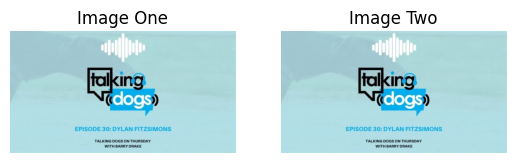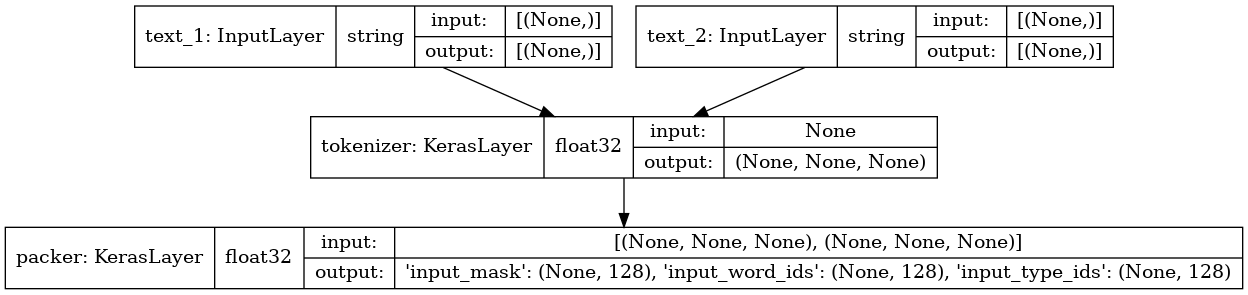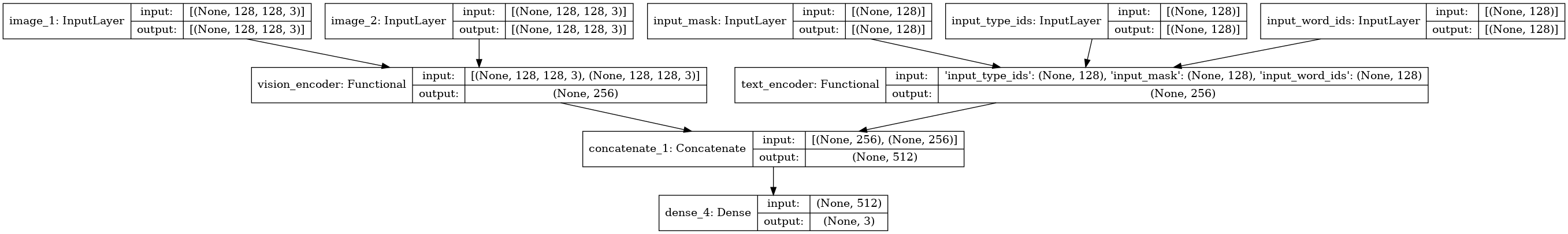Code examples / Natural Language Processing / Multimodal entailment

# Multimodal entailment

Author: Sayak Paul
Date created: 2021/08/08
Description: Training a multimodal model for predicting entailment.

ⓘ This example uses Keras 2View in ColabGitHub source

## Introduction

In this example, we will build and train a model for predicting multimodal entailment. We will be using the multimodal entailment dataset recently introduced by Google Research.

### What is multimodal entailment?

On social media platforms, to audit and moderate content we may want to find answers to the following questions in near real-time:

• Does a given piece of information contradict the other?
• Does a given piece of information imply the other?

In NLP, this task is called analyzing textual entailment. However, that's only when the information comes from text content. In practice, it's often the case the information available comes not just from text content, but from a multimodal combination of text, images, audio, video, etc. Multimodal entailment is simply the extension of textual entailment to a variety of new input modalities.

### Requirements

This example requires TensorFlow 2.5 or higher. In addition, TensorFlow Hub and TensorFlow Text are required for the BERT model (Devlin et al.). These libraries can be installed using the following command:

``````!pip install -q tensorflow_text
``````

## Imports

``````from sklearn.model_selection import train_test_split
import matplotlib.pyplot as plt
import pandas as pd
import numpy as np
import os

import tensorflow as tf
import tensorflow_hub as hub
import tensorflow_text as text
from tensorflow import keras
``````

## Define a label map

``````label_map = {"Contradictory": 0, "Implies": 1, "NoEntailment": 2}
``````

## Collect the dataset

The original dataset is available here. It comes with URLs of images which are hosted on Twitter's photo storage system called the Photo Blob Storage (PBS for short). We will be working with the downloaded images along with additional data that comes with the original dataset. Thanks to Nilabhra Roy Chowdhury who worked on preparing the image data.

``````image_base_path = keras.utils.get_file(
"tweet_images",
untar=True,
)
``````

## Read the dataset and apply basic preprocessing

``````df = pd.read_csv(
"https://github.com/sayakpaul/Multimodal-Entailment-Baseline/raw/main/csvs/tweets.csv"
)
df.sample(10)
``````
id_1 text_1 image_1 id_2 text_2 image_2 label
291 1330800194863190016 #KLM1167 (B738): #AMS (Amsterdam) to #HEL (Van... http://pbs.twimg.com/media/EnfzuZAW4AE236p.png 1378695438480588802 #CKK205 (B77L): #PVG (Shanghai) to #AMS (Amste... http://pbs.twimg.com/media/EyIcMexXEAE6gia.png NoEntailment
37 1366581728312057856 Friends, interested all go to have a look!\n@j... http://pbs.twimg.com/media/EvcS1v4UcAEEXPO.jpg 1373810535066570759 Friends, interested all go to have a look!\n@f... http://pbs.twimg.com/media/ExDBZqwVIAQ4LWk.jpg Contradictory
315 1352551603258052608 #WINk Drops I have earned today🚀\n\nToday:1/22... http://pbs.twimg.com/media/EsTdcLLVcAIiFKT.jpg 1354636016234098688 #WINk Drops I have earned today☀\n\nToday:1/28... http://pbs.twimg.com/media/EsyhK-qU0AgfMAH.jpg NoEntailment
146 1340185132293099523 I know sometimes I am weird to you.\n\nBecause... http://pbs.twimg.com/media/EplLRriWwAAJ2AE.jpg 1359755419883814913 I put my sword down and get on my knees to swe... http://pbs.twimg.com/media/Et7SWWeWYAICK-c.jpg NoEntailment
1351 1381256604926967813 Finally completed the skin rendering. Will sta... http://pbs.twimg.com/media/Eys1j7NVIAgF-YF.jpg 1381630932092784641 Hair rendering. Will finish the hair by tomorr... http://pbs.twimg.com/media/EyyKAoaUUAElm-e.jpg NoEntailment
368 1371883298805403649 📉 \$LINK Number of Receiving Addresses (7d MA) ... http://pbs.twimg.com/media/EwnoltOWEAAS4mG.jpg 1373216720974979072 📉 \$LINK Number of Receiving Addresses (7d MA) ... http://pbs.twimg.com/media/Ew6lVGYXEAE6Ugi.jpg NoEntailment
1112 1377679115159887873 April is National Distracted Driving Awareness... http://pbs.twimg.com/media/Ex5_u7UVIAARjQ2.jpg 1379075258448281608 April is Distracted Driving Awareness Month. ... http://pbs.twimg.com/media/EyN1YjpWUAMc5ak.jpg NoEntailment
264 1330727515741167619 ♥️Verse Of The Day♥️\n.\n#VerseOfTheDay #Quran... http://pbs.twimg.com/media/EnexnydXIAYuI11.jpg 1332623263495819264 ♥️Verse Of The Day♥️\n.\n#VerseOfTheDay #Quran... http://pbs.twimg.com/media/En5ty1VXUAATALP.jpg NoEntailment
865 1377784616275296261 No white picket fence can keep us in. #TBT 200... http://pbs.twimg.com/media/Ex7fzouWQAITAq8.jpg 1380175915804672012 Sometimes you just need to change your altitud... http://pbs.twimg.com/media/EydernQXIAk2g5v.jpg NoEntailment

The columns we are interested in are the following:

• `text_1`
• `image_1`
• `text_2`
• `image_2`
• `label`

The entailment task is formulated as the following:

Given the pairs of (`text_1`, `image_1`) and (`text_2`, `image_2`) do they entail (or not entail or contradict) each other?

We have the images already downloaded. `image_1` is downloaded as `id1` as its filename and `image2` is downloaded as `id2` as its filename. In the next step, we will add two more columns to `df` - filepaths of `image_1`s and `image_2`s.

``````images_one_paths = []
images_two_paths = []

for idx in range(len(df)):
current_row = df.iloc[idx]
id_1 = current_row["id_1"]
id_2 = current_row["id_2"]
extentsion_one = current_row["image_1"].split(".")[-1]
extentsion_two = current_row["image_2"].split(".")[-1]

image_one_path = os.path.join(image_base_path, str(id_1) + f".{extentsion_one}")
image_two_path = os.path.join(image_base_path, str(id_2) + f".{extentsion_two}")

images_one_paths.append(image_one_path)
images_two_paths.append(image_two_path)

df["image_1_path"] = images_one_paths
df["image_2_path"] = images_two_paths

# Create another column containing the integer ids of
# the string labels.
df["label_idx"] = df["label"].apply(lambda x: label_map[x])
``````

## Dataset visualization

``````def visualize(idx):
current_row = df.iloc[idx]
text_1 = current_row["text_1"]
text_2 = current_row["text_2"]
label = current_row["label"]

plt.subplot(1, 2, 1)
plt.imshow(image_1)
plt.axis("off")
plt.title("Image One")
plt.subplot(1, 2, 2)
plt.imshow(image_1)
plt.axis("off")
plt.title("Image Two")
plt.show()

print(f"Text one: {text_1}")
print(f"Text two: {text_2}")
print(f"Label: {label}")

random_idx = np.random.choice(len(df))
visualize(random_idx)

random_idx = np.random.choice(len(df))
visualize(random_idx)
````````````Text one: Friends, interested all go to have a look!
@ThePartyGoddess @OurLadyAngels @BJsWholesale @Richard_Jeni @FashionLavidaG @RapaRooski @DMVTHING @DeMarcoReports @LobidaFo @DeMarcoMorgan https://t.co/cStULl7y7G
Text two: Friends, interested all go to have a look!
@smittyses @CYosabel @crum_7 @CrumDarrell @ElymalikU @jenloarn @SoCodiePrevost @roblowry82 @Crummy_14 @CSchmelzenbach https://t.co/IZphLTNzgl
````````````Text one: 👟 KICK OFF @ MARDEN SPORTS COMPLEX
``````
``````We're underway in the Round 6 opener!
``````
``````📺: @Foxtel, @kayosports
📱: My Football Live app https://t.co/wHSpvQaoGC
``````
``````#WLeague #ADLvMVC #AUFC #MVFC https://t.co/3Smp8KXm8W
Text two: 👟 KICK OFF @ MARSDEN SPORTS COMPLEX
``````
``````We're underway in sunny Adelaide!
``````
``````📺: @Foxtel, @kayosports
📱: My Football Live app https://t.co/wHSpvQaoGC
``````
``````#ADLvCBR #WLeague #AUFC #UnitedAlways https://t.co/fG1PyLQXM4
Label: NoEntailment
``````

## Train/test split

The dataset suffers from class imbalance problem. We can confirm that in the following cell.

``````df["label"].value_counts()
``````
``````NoEntailment     1182
Implies           109
Name: label, dtype: int64
``````

To account for that we will go for a stratified split.

``````# 10% for test
train_df, test_df = train_test_split(
df, test_size=0.1, stratify=df["label"].values, random_state=42
)
# 5% for validation
train_df, val_df = train_test_split(
train_df, test_size=0.05, stratify=train_df["label"].values, random_state=42
)

print(f"Total training examples: {len(train_df)}")
print(f"Total validation examples: {len(val_df)}")
print(f"Total test examples: {len(test_df)}")
``````
``````Total training examples: 1197
Total validation examples: 63
Total test examples: 140
``````

## Data input pipeline

TensorFlow Hub provides variety of BERT family of models. Each of those models comes with a corresponding preprocessing layer. You can learn more about these models and their preprocessing layers from this resource.

To keep the runtime of this example relatively short, we will use a smaller variant of the original BERT model.

``````# Define TF Hub paths to the BERT encoder and its preprocessor
bert_model_path = (
"https://tfhub.dev/tensorflow/small_bert/bert_en_uncased_L-2_H-256_A-4/1"
)
bert_preprocess_path = "https://tfhub.dev/tensorflow/bert_en_uncased_preprocess/3"
``````

Our text preprocessing code mostly comes from this tutorial. You are highly encouraged to check out the tutorial to learn more about the input preprocessing.

``````def make_bert_preprocessing_model(sentence_features, seq_length=128):
"""Returns Model mapping string features to BERT inputs.

Args:
sentence_features: A list with the names of string-valued features.
seq_length: An integer that defines the sequence length of BERT inputs.

Returns:
A Keras Model that can be called on a list or dict of string Tensors
(with the order or names, resp., given by sentence_features) and
returns a dict of tensors for input to BERT.
"""

input_segments = [
tf.keras.layers.Input(shape=(), dtype=tf.string, name=ft)
for ft in sentence_features
]

# Tokenize the text to word pieces.
tokenizer = hub.KerasLayer(bert_preprocess.tokenize, name="tokenizer")
segments = [tokenizer(s) for s in input_segments]

# Optional: Trim segments in a smart way to fit seq_length.
# Simple cases (like this example) can skip this step and let
# the next step apply a default truncation to approximately equal lengths.
truncated_segments = segments

# Pack inputs. The details (start/end token ids, dict of output tensors)
# are model-dependent, so this gets loaded from the SavedModel.
packer = hub.KerasLayer(
bert_preprocess.bert_pack_inputs,
arguments=dict(seq_length=seq_length),
name="packer",
)
model_inputs = packer(truncated_segments)
return keras.Model(input_segments, model_inputs)

bert_preprocess_model = make_bert_preprocessing_model(["text_1", "text_2"])
keras.utils.plot_model(bert_preprocess_model, show_shapes=True, show_dtype=True)
``````### Run the preprocessor on a sample input

``````idx = np.random.choice(len(train_df))
row = train_df.iloc[idx]
sample_text_1, sample_text_2 = row["text_1"], row["text_2"]
print(f"Text 1: {sample_text_1}")
print(f"Text 2: {sample_text_2}")

test_text = [np.array([sample_text_1]), np.array([sample_text_2])]
text_preprocessed = bert_preprocess_model(test_text)

print("Keys           : ", list(text_preprocessed.keys()))
print("Shape Word Ids : ", text_preprocessed["input_word_ids"].shape)
print("Word Ids       : ", text_preprocessed["input_word_ids"][0, :16])
print("Shape Type Ids : ", text_preprocessed["input_type_ids"].shape)
print("Type Ids       : ", text_preprocessed["input_type_ids"][0, :16])
``````
``````Text 1: Renewables met 97% of Scotland's electricity demand in 2020!!!!
https://t.co/wi5c9UFAUF https://t.co/arcuBgh0BP
Text 2: Renewables met 97% of Scotland's electricity demand in 2020 https://t.co/SrhyqPnIkU https://t.co/LORgvTM7Sn
Shape Word Ids :  (1, 128)
Word Ids       :  tf.Tensor(
[  101 13918  2015  2777  5989  1003  1997  3885  1005  1055  6451  5157
1999 12609   999   999], shape=(16,), dtype=int32)
Input Mask     :  tf.Tensor([1 1 1 1 1 1 1 1 1 1 1 1 1 1 1 1], shape=(16,), dtype=int32)
Shape Type Ids :  (1, 128)
Type Ids       :  tf.Tensor([0 0 0 0 0 0 0 0 0 0 0 0 0 0 0 0], shape=(16,), dtype=int32)
``````

We will now create `tf.data.Dataset` objects from the dataframes.

Note that the text inputs will be preprocessed as a part of the data input pipeline. But the preprocessing modules can also be a part of their corresponding BERT models. This helps reduce the training/serving skew and lets our models operate with raw text inputs. Follow this tutorial to learn more about how to incorporate the preprocessing modules directly inside the models.

``````def dataframe_to_dataset(dataframe):
columns = ["image_1_path", "image_2_path", "text_1", "text_2", "label_idx"]
dataframe = dataframe[columns].copy()
labels = dataframe.pop("label_idx")
ds = tf.data.Dataset.from_tensor_slices((dict(dataframe), labels))
ds = ds.shuffle(buffer_size=len(dataframe))
return ds
``````

### Preprocessing utilities

``````resize = (128, 128)

def preprocess_image(image_path):
extension = tf.strings.split(image_path)[-1]

if extension == b"jpg":
image = tf.image.decode_jpeg(image, 3)
else:
image = tf.image.decode_png(image, 3)
image = tf.image.resize(image, resize)
return image

def preprocess_text(text_1, text_2):
text_1 = tf.convert_to_tensor([text_1])
text_2 = tf.convert_to_tensor([text_2])
output = bert_preprocess_model([text_1, text_2])
output = {feature: tf.squeeze(output[feature]) for feature in bert_input_features}
return output

def preprocess_text_and_image(sample):
image_1 = preprocess_image(sample["image_1_path"])
image_2 = preprocess_image(sample["image_2_path"])
text = preprocess_text(sample["text_1"], sample["text_2"])
return {"image_1": image_1, "image_2": image_2, "text": text}
``````

### Create the final datasets

``````batch_size = 32
auto = tf.data.AUTOTUNE

def prepare_dataset(dataframe, training=True):
ds = dataframe_to_dataset(dataframe)
if training:
ds = ds.shuffle(len(train_df))
ds = ds.map(lambda x, y: (preprocess_text_and_image(x), y)).cache()
ds = ds.batch(batch_size).prefetch(auto)
return ds

train_ds = prepare_dataset(train_df)
validation_ds = prepare_dataset(val_df, False)
test_ds = prepare_dataset(test_df, False)
``````

## Model building utilities

Our final model will accept two images along with their text counterparts. While the images will be directly fed to the model the text inputs will first be preprocessed and then will make it into the model. Below is a visual illustration of this approach:The model consists of the following elements:

• A standalone encoder for the images. We will use a ResNet50V2 pre-trained on the ImageNet-1k dataset for this.
• A standalone encoder for the images. A pre-trained BERT will be used for this.

After extracting the individual embeddings, they will be projected in an identical space. Finally, their projections will be concatenated and be fed to the final classification layer.

This is a multi-class classification problem involving the following classes:

• NoEntailment
• Implies

`project_embeddings()`, `create_vision_encoder()`, and `create_text_encoder()` utilities are referred from this example.

Projection utilities

``````def project_embeddings(
embeddings, num_projection_layers, projection_dims, dropout_rate
):
projected_embeddings = keras.layers.Dense(units=projection_dims)(embeddings)
for _ in range(num_projection_layers):
x = tf.nn.gelu(projected_embeddings)
x = keras.layers.Dense(projection_dims)(x)
x = keras.layers.Dropout(dropout_rate)(x)
projected_embeddings = keras.layers.LayerNormalization()(x)
return projected_embeddings
``````

Vision encoder utilities

``````def create_vision_encoder(
num_projection_layers, projection_dims, dropout_rate, trainable=False
):
# Load the pre-trained ResNet50V2 model to be used as the base encoder.
resnet_v2 = keras.applications.ResNet50V2(
include_top=False, weights="imagenet", pooling="avg"
)
# Set the trainability of the base encoder.
for layer in resnet_v2.layers:
layer.trainable = trainable

# Receive the images as inputs.
image_1 = keras.Input(shape=(128, 128, 3), name="image_1")
image_2 = keras.Input(shape=(128, 128, 3), name="image_2")

# Preprocess the input image.
preprocessed_1 = keras.applications.resnet_v2.preprocess_input(image_1)
preprocessed_2 = keras.applications.resnet_v2.preprocess_input(image_2)

# Generate the embeddings for the images using the resnet_v2 model
# concatenate them.
embeddings_1 = resnet_v2(preprocessed_1)
embeddings_2 = resnet_v2(preprocessed_2)
embeddings = keras.layers.Concatenate()([embeddings_1, embeddings_2])

# Project the embeddings produced by the model.
outputs = project_embeddings(
embeddings, num_projection_layers, projection_dims, dropout_rate
)
# Create the vision encoder model.
return keras.Model([image_1, image_2], outputs, name="vision_encoder")
``````

Text encoder utilities

``````def create_text_encoder(
num_projection_layers, projection_dims, dropout_rate, trainable=False
):
# Load the pre-trained BERT model to be used as the base encoder.
bert = hub.KerasLayer(bert_model_path, name="bert",)
# Set the trainability of the base encoder.
bert.trainable = trainable

# Receive the text as inputs.
inputs = {
feature: keras.Input(shape=(128,), dtype=tf.int32, name=feature)
for feature in bert_input_features
}

# Generate embeddings for the preprocessed text using the BERT model.
embeddings = bert(inputs)["pooled_output"]

# Project the embeddings produced by the model.
outputs = project_embeddings(
embeddings, num_projection_layers, projection_dims, dropout_rate
)
# Create the text encoder model.
return keras.Model(inputs, outputs, name="text_encoder")
``````

Multimodal model utilities

``````def create_multimodal_model(
num_projection_layers=1,
projection_dims=256,
dropout_rate=0.1,
vision_trainable=False,
text_trainable=False,
):
# Receive the images as inputs.
image_1 = keras.Input(shape=(128, 128, 3), name="image_1")
image_2 = keras.Input(shape=(128, 128, 3), name="image_2")

# Receive the text as inputs.
text_inputs = {
feature: keras.Input(shape=(128,), dtype=tf.int32, name=feature)
for feature in bert_input_features
}

# Create the encoders.
vision_encoder = create_vision_encoder(
num_projection_layers, projection_dims, dropout_rate, vision_trainable
)
text_encoder = create_text_encoder(
num_projection_layers, projection_dims, dropout_rate, text_trainable
)

# Fetch the embedding projections.
vision_projections = vision_encoder([image_1, image_2])
text_projections = text_encoder(text_inputs)

# Concatenate the projections and pass through the classification layer.
concatenated = keras.layers.Concatenate()([vision_projections, text_projections])
outputs = keras.layers.Dense(3, activation="softmax")(concatenated)
return keras.Model([image_1, image_2, text_inputs], outputs)

multimodal_model = create_multimodal_model()
keras.utils.plot_model(multimodal_model, show_shapes=True)
``````You can inspect the structure of the individual encoders as well by setting the `expand_nested` argument of `plot_model()` to `True`. You are encouraged to play with the different hyperparameters involved in building this model and observe how the final performance is affected.

## Compile and train the model

``````multimodal_model.compile(
)

history = multimodal_model.fit(train_ds, validation_data=validation_ds, epochs=10)
``````
``````Epoch 1/10
38/38 [==============================] - 49s 789ms/step - loss: 1.0014 - accuracy: 0.8229 - val_loss: 0.5514 - val_accuracy: 0.8571
Epoch 2/10
38/38 [==============================] - 3s 90ms/step - loss: 0.4019 - accuracy: 0.8814 - val_loss: 0.5866 - val_accuracy: 0.8571
Epoch 3/10
38/38 [==============================] - 3s 90ms/step - loss: 0.3557 - accuracy: 0.8897 - val_loss: 0.5929 - val_accuracy: 0.8571
Epoch 4/10
38/38 [==============================] - 3s 91ms/step - loss: 0.2877 - accuracy: 0.9006 - val_loss: 0.6272 - val_accuracy: 0.8571
Epoch 5/10
38/38 [==============================] - 3s 91ms/step - loss: 0.1796 - accuracy: 0.9398 - val_loss: 0.8545 - val_accuracy: 0.8254
Epoch 6/10
38/38 [==============================] - 3s 91ms/step - loss: 0.1292 - accuracy: 0.9566 - val_loss: 1.2276 - val_accuracy: 0.8413
Epoch 7/10
38/38 [==============================] - 3s 91ms/step - loss: 0.1015 - accuracy: 0.9666 - val_loss: 1.2914 - val_accuracy: 0.7778
Epoch 8/10
38/38 [==============================] - 3s 92ms/step - loss: 0.1253 - accuracy: 0.9524 - val_loss: 1.1944 - val_accuracy: 0.8413
Epoch 9/10
38/38 [==============================] - 3s 92ms/step - loss: 0.3064 - accuracy: 0.9131 - val_loss: 1.2162 - val_accuracy: 0.8095
Epoch 10/10
38/38 [==============================] - 3s 92ms/step - loss: 0.2212 - accuracy: 0.9248 - val_loss: 1.1080 - val_accuracy: 0.8413
``````

## Evaluate the model

``````_, acc = multimodal_model.evaluate(test_ds)
print(f"Accuracy on the test set: {round(acc * 100, 2)}%.")
``````
``````5/5 [==============================] - 6s 1s/step - loss: 0.8390 - accuracy: 0.8429
Accuracy on the test set: 84.29%.
``````

Incorporating regularization:

The training logs suggest that the model is starting to overfit and may have benefitted from regularization. Dropout (Srivastava et al.) is a simple yet powerful regularization technique that we can use in our model. But how should we apply it here?

We could always introduce Dropout (`keras.layers.Dropout`) in between different layers of the model. But here is another recipe. Our model expects inputs from two different data modalities. What if either of the modalities is not present during inference? To account for this, we can introduce Dropout to the individual projections just before they get concatenated:

``````vision_projections = keras.layers.Dropout(rate)(vision_projections)
text_projections = keras.layers.Dropout(rate)(text_projections)
concatenated = keras.layers.Concatenate()([vision_projections, text_projections])
``````

Attending to what matters:

Do all parts of the images correspond equally to their textual counterparts? It's likely not the case. To make our model only focus on the most important bits of the images that relate well to their corresponding textual parts we can use "cross-attention":

``````# Embeddings.
vision_projections = vision_encoder([image_1, image_2])
text_projections = text_encoder(text_inputs)

# Cross-attention (Luong-style).
query_value_attention_seq = keras.layers.Attention(use_scale=True, dropout=0.2)(
[vision_projections, text_projections]
)
# Concatenate.
concatenated = keras.layers.Concatenate()([vision_projections, text_projections])
contextual = keras.layers.Concatenate()([concatenated, query_value_attention_seq])
``````

To see this in action, refer to this notebook.

Handling class imbalance:

The dataset suffers from class imbalance. Investigating the confusion matrix of the above model reveals that it performs poorly on the minority classes. If we had used a weighted loss then the training would have been more guided. You can check out this notebook that takes class-imbalance into account during model training.

Using only text inputs:

Also, what if we had only incorporated text inputs for the entailment task? Because of the nature of the text inputs encountered on social media platforms, text inputs alone would have hurt the final performance. Under a similar training setup, by only using text inputs we get to 67.14% top-1 accuracy on the same test set. Refer to this notebook for details.

Finally, here is a table comparing different approaches taken for the entailment task:

Type Standard
Cross-entropy
Loss-weighted
Cross-entropy
Focal Loss
Multimodal 77.86% 67.86% 86.43%
Only text 67.14% 11.43% 37.86%

You can check out this repository to learn more about how the experiments were conducted to obtain these numbers.

## Final remarks

• The architecture we used in this example is too large for the number of data points available for training. It's going to benefit from more data.
• We used a smaller variant of the original BERT model. Chances are high that with a larger variant, this performance will be improved. TensorFlow Hub provides a number of different BERT models that you can experiment with.
• We kept the pre-trained models frozen. Fine-tuning them on the multimodal entailment task would could resulted in better performance.
• We built a simple baseline model for the multimodal entailment task. There are various approaches that have been proposed to tackle the entailment problem. This presentation deck from the Recognizing Multimodal Entailment tutorial provides a comprehensive overview.

You can use the trained model hosted on Hugging Face Hub and try the demo on Hugging Face Spaces